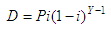# Calculate Depreciation

In this article we’re going to calculate depreciation by implementing two relevant formulas: rate and amount.

### 1. Depreciation Rate

This code calculates the annual depreciation rate of an investment. You must provide the original price of the item, its resale price, and its age in years.

The depreciation rate is calculated by the following formula:where:
D = depreciation rate
T = resale price
P = original price
Y = age

This is our simple Matlab code to calculate the above formula:

function dr = depreciation_rate(p,t,y)
dr = 100*(1-(t/p)^(1/y));

We create another script to test and drive the above m-file:

clc, clear, format compact, format bank

p = input('Enter original price: ');
t = input(
'Enter resale price: ');
y = input(
'Enter number of years: ');

dr = depreciation_rate(p,t,y)

Example:

Jill bought her car for \$4933.76 and sold it for \$2400 three years later. What was its actual depreciation rate?

We launch our helpful code, and get:

Enter original price: 4933.76
Enter resale price: 2400
Enter number of years: 3

The result is:

dr = 21.35 (%)

### 2. Depreciation Amount

This section calculates the amount depreciated within a given year for a depreciating investment. You must provide the original price of the investment, its depreciation rate, and the year of depreciation.

The depreciation amount is claulated by the following formula:where:
D = depreciation amount
P = original price
i = depreciation rate
Y = year of depreciation

This is our simple Matlab code to calculate the above formula:

function da = depreciation_amount(p,i,y)
i = i/100;
da = p*i*(1-i)^(y-1);

We create another script to test and drive the above m-file:

clc, clear, format compact, format bank

p = input('Enter original price: ');
i = input(
'Enter depreciation rate: ');
y = input(
'Enter year of depreciation: ');

da = depreciation_amount(p,i,y)

Example:

Jill bought her car for \$4933.76. Her model car depreciates at an average annual rate of 21%. What amount has the car depreciated in the first year?

We now launch our also helpful new code...and...

Enter original price: 4933.76
Enter depreciation rate: 21
Enter year of depreciation: 1

The result is:

da = 1036.09

From 'Calculate Depreciation' to home

From 'Calculate Depreciation' to 'Finance Formulas'